## Differential equations gone wrong

There was a post at WUWT called A simple demonstration of chaos and unreliability of computer models. It puported to solve a simple problem of a radiating black body coming to equilibrium with an internal heat source. But it was formulated as a non-linear recurrence relation. Some chaotic behaviour was demonstrated, but mis-attributed to methods of evaluating powers, and floating point issues.

I commented, first taking issue with the foolishness of this line of posting which quotes a simple and easily solved issue to say that there is something wrong with computer models in general. By which they mean, of course, climate models, but the alleged problem is quite generic. If the proposition were accepted, large areas of much-used engineering maths would have to be abandoned. Which is nonsense. David Evans claiming some issue about partial derivatives was another example. Also Hans Jelbring.

I went on to talk about the fact that the recurrence relation did not at all solve the underlying differential equation (DE). I made reference to stiff differential equations and suggested ways in which this could be improved. In a way, this missed the mark, because the author had never formulated it as a de. He just wrote down the recurrence for what was a very large time step, and found fault with it. But the recurrence does not in itself describe any real physics. It only does so insofar as it provides approximate solutions to the differential equation which does represent the process. And in his case, it certainly didn't. Because of the excessive timestep, the solutions were oscillatory, instead of the proper exponential approach to equilibrium. In one case, there was still convergence, in the other not. The latter case did show chaotic behaviour, where he got lost with red herrings about floating point and how powers are calculated. This was reflected in the discussion. In fact, the relevant maths is the magnification of small differences, which is the key point of chaos. The source of the differences is immaterial. But the magnification is already present in the linearised equation.

So in this post, I'll talk more about the differential equations aspect. Posters at WUWT frequently have no concept of what is involved in numerical DE. But there was also a real chaos problem. It's not connected with any physics here, but it is an example of the same kind as the standard quadratic recurrence, often related to predator-prey, and described at WUWT here. I think I can use this as a useful example of the chaotic aspect of climate modelling - how dependence on initial conditions fails, but this doesn't affect the ability to model climate. That will be Part 2 (probably more interesting).

#### Differential equations

Some theory to start with, but if you're just interested in the WUWT example, you can skim to the last heading. A general DE takes the form
y'=dy/dt=F(y,t)
where y and F can both be vectors (of equal order). You may say, but what about higher order de's? They can be converted to first order by adding extra variables and equations. For example, y'=y1, y1'=y2 etc.

You can integrate a DE over timesteps:
y1-y0=∫F(y,t)dt ... over range t0 to t1

Of course, the catch is that you don't know the y values in the integrand until you have solved. But you can approximate. The simplest is to assume that F(y,t)=F(y0,t0) through the timestep. This is Euler's method.

#### Numerical integration

Euler's method gives the recurrence:

yn+1-yn=F(yn,tn) dt ... dt=tn+1-tn

I'm assuming here a solution from initial values ie specified y0.

Mostly, it gives first order accuracy as dt→0; that is, the approximate solution will deviate from the exact by O(dt). So it works for small dt, when the starting value is a fair approx for the short interval.

You can improve by better approximating the integral. Stable methods tend to use the most current data, including yn+1. You can either use a linear dependence and solve the resulting linear equation for yn+1, or you can use a predictor-corrector method, where you guess yn+1 and then solve again to improve the guess. You can use more past points (multi-step) to get higher order integration, or use a bootstrapping method (Runge-Kutta) with intermediate points. This theory is more than a century old, and is the minimum that practitioners need to know

#### Stability

You'll hear a lot about stability. There is an important and special kind of instability in de solution. An n-th order linear system (n first order equations in our convention) has n independent solutions, which can be linearly combined. Non-linear equations usually behave like this locally. A solution procedure will potentially generate all of them, depending on initial conditions. If you set conditions for one solution, but there is another solution that grows faster, then any error will be interpreted as a component of that (and other) solution, and will grow, eventually destroying the solution you are following.

For example, the equation y''=y has solutions exp(t), exp(-t). If you set y(0)=1, y'(0)=-1, you would be hoping to track (for t>0) exp(-t), which diminishes. But any errors will introduce a component of exp(t), which will grow and swamp your solution.

These considerations also apply to the approximating recurrence relations. A very common problem is that the approximation of a DE allows a growing solution which wasn't a problem in the exact set.

But for now, I'll talk about a more primitive stability. A simple equation is:
y' = -y
This has solution exp(-t), with condition y(0)=1. By Euler's method, the recurrence
yn+1-yn=-yn dt
which has exact solution
yn=(1-dt)n

For small dt, this diminishes smoothly and geometrically, and is a good approximation to the exponential. But for dt = 1, it drops immediately to 0 and stays there. That is not too bad, since 0 is the true limit, bit it isn't getting there in the right style. And worse, if dt>1, it starts to oscillate while converging.

But worse still, if dt>2, the oscillations grow rather than diminish. And small differences will be amplified at the same rate. That isn't true instability - the solution range just expands uniformly. Update - apologies, I has mixed up links and pointed to the wrong graph here - fixed.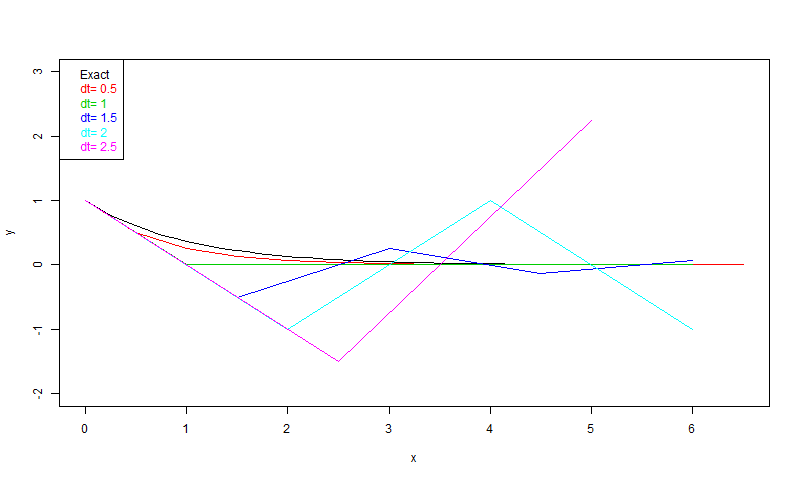It's clear that to get a decent solution, you need a timestep a lot less than the time constant (=1) of the exponential.

#### Solving WUWT style

So back to the WUWT example. It's solving for approach to equilibium of a black body with constant heat source, and S-B radiation. It's never actually formulated there as a DE; the author goes straight to iterations:

Tn+1=Tn+b*(1-(Tn/Te)4)

where Te is the equilibrium temperature (243 K) and b (his β) incorporates specific heat and time step. But it is the Euler solution. With his lower b=100, you can already see what is wrong. He starts with T0=300K, so (T0/Te)4= 2.32, and T drops to 168K during the first step. But he is assuming radiation at 300K throughout that step.

Here is a plot of some reasonable attempts to solve the equation. The time constant of the linearised equation is Te/4=60.75.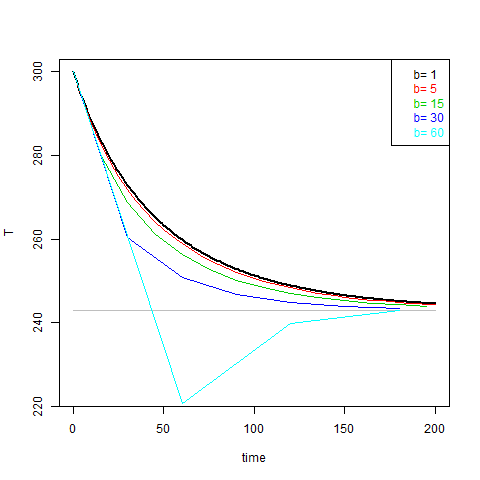Already b>15 is looking doubtful, and b=60 (=time constant) is wrong. But here are the "solutions" in the WUWT post: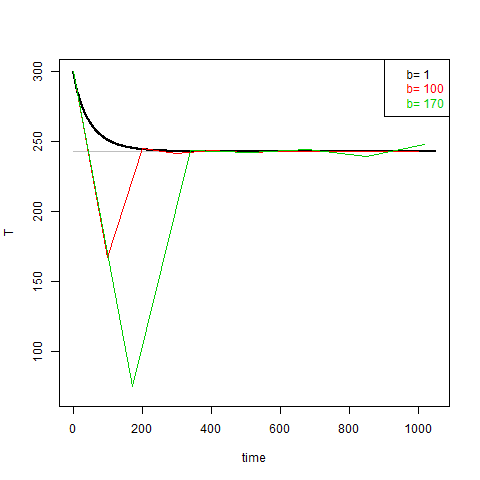Clearly they look nothing like the correct black curve. It is true that, somewhat coincidentally, they approach the equilibrium value on the second step. The b=100 solution then converges, with oscillation. That is because the timestep is less than twice the time constant (see first plot). But b=170 is more than twice time constant, and the sequence diverges away from equilibrium.

This behaviour was noted at WUWT, and also that b=100 was stable, whereas b=170 led to different results after a hundred timesteps or so, depending on how the fourth power was calculated. The reason for that is that the growing solutions magnify any small discrepancy - from anywhere. With linear equations, that wouldn't matter much, because the actual solutions would also expand. But here the non-linearity prevents that. If the body gets to 486K, for example, it will emit 16 times the heat source amount. So in effect, the trajectories get bounced back. But this doesn't overcome the growth in small differences. They continue almost as if there were linear growth.

In fact, the rate of growth of error can be roughly approximated as 1-b/Tc per step, where the time contant Tc=T0/4=60.75. In magnitude, with b=170 it is (-1.8)^n where n is number of timesteps. For n=70, where WUWT found errors affecting 4th decimal place, the magnification is 7.4E17. That would make a big deal out of any floating poiint innacuracy at the start. I say the approx is rough because it is the rate of growth of the linear equation near equilibrium - generally the effect of non-linearity will be to reduce it.

As said, none of this has anything to do with how a competent practitioner would solve the physical problem. It doesn't show any problem for models, climate or otherwise. But it does create an artificial chaotic process, which I'll talk about in the next post.

#### Appendix - a better method

I said above and at WUWT that there are many better methods than forward Euler, and they go back more than a century. I specifically mentioned trapezoidal. In PDE, this is called Crank-Nicolson. Instead of assuming the initial value of F for the timestep integration, a linear approximation is assumed. Or equivalently, a constant approx with the mid-point value.

This could be done just with higher derivatives from the start point. But a better way is to make it linearly dependent on the unknown end-step value. This is implicitness, and gives more stability. The mid-point approx is
F(T0)+.5*F'(T0)*(T1-T0), where F(T)=b*(1-(T/Te)4)
If there were dependence on t, that would need another partial derivative, but there isn't here.

Sorting out the algebra makes the recurrence still first order:

Tn+1=Tn+F(Tn)/(1-F'(Tn)/2)

Here are the plots. I show side by side the Euler and trapezoidal. First the reasonable timestep case: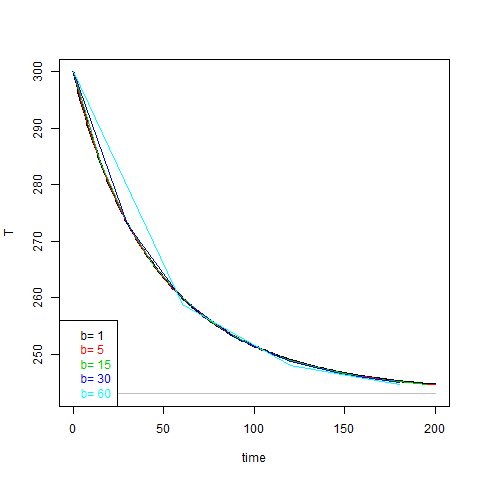The trapezoidal is clearly more accurate, even up to timestep equal to the time constant. In fact it is more accurate than it looks with the line drawing. Though the linear interpolate deviates, the actual drawn points are very close to the curve.

And now the long WUWT timesteps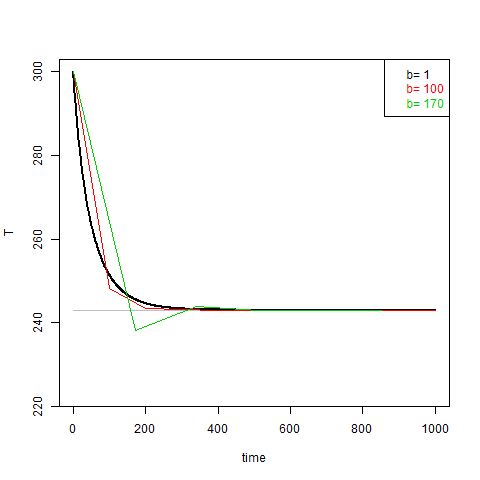Again, the situation is much better. Oscillations are very small, and there is no instability. However, as expected, the solutions can't be accurate when a single timestep covers a large fraction of the exponential descent.

1.How incompetent does one have to be to miscode and mischaracterize a uniform idealized body approaching steady-state thermal equilibrium with its surroundings via radiative exchange? Or to realize that the physical result will be an inherently non-chaotic monotonic approach to a limiting value and that if there is any overshoot or undershoot in the iterative numerical solution it was because of a poor algorithm or bad coding?

But then to write a public note about it (one that WUWT thought worth posting) and garner over 200 comments? Amateur crankery at its finest. At least more of the comments than usual pointed out the errors, including those from you, Joel D. Shore, 'Mike', Dan Hughes and possibly others. I'm not going to wade through them all.

1.Magma, I paid no attention to this WUWT post (as with most of them) but what you point out has to be the most jaw-dropping example of conspiracy numerology I have come across. We have all heard of Applied Math but this is what I would term Misapplied Math.

There was no point in Nick going through what he did, and I hate to see him waste his time trying to correct the WUWT poster. What we really have to do in these cases is act like a typical University professor. Take a screenshot of the offending article and then Photoshop a Big Red X across it.

2.If only that could be done... quick, who's in charge of the Internet? I've been looking for that guy's email for years.

There was Wolfgang Pauli's "Das ist nicht nur nicht richtig, es ist nicht einmal falsch!" rendered more snappily as "Not even wrong." Or consider an observation by Isaac Asimov, summarized as "wronger than wrong" here: http://www.scientificamerican.com/article/wronger-than-wrong/

2.If a computer model is reliable or not depends on the programmer.
If one makes a single beginner's mistake, the computer model may not be reliable.

But demonstrating that one can make a bunch of beginner's mistakes, does not hint or prove that computer models without beginner's mistakes are unreliable.

3.The second thing they teach you after Eulers method is to double the size of the step and run it again. If the answer is significantly different your step size was too large. Rinse and repeat with smaller step.

These guys are numerically suicidal.

1.Yup, and/or half the step size in your algorithm, back in the day (35 years ago) it was FD with RK4 and adjustable step sizing or FEA with appropriate mesh generation. I always checked my models for potential instability issues, always.

4.Nick- thanks for putting the time into this. It's a compact, self-contained example of the basic incompetence of denialist criticism of climate modelling. They don't really know what they are talking about, and are too dim to realise it, ie the Dunning-Kruger effect.

The next time someone says to me 'oh, but the denialists say they have proof the models are wrong', I'll use this example to demonstrate that (amongst their other other climate-related scientific shortcomings) they are mathematically clueless.

5.I forgot to say: thank you for writing this up.

1.Thanks for the suggestion. The chaos part turned out to be quite interesting, if nothing to do with the de.